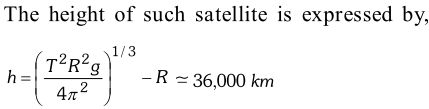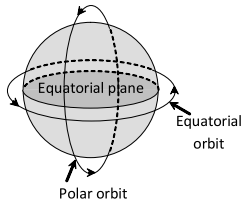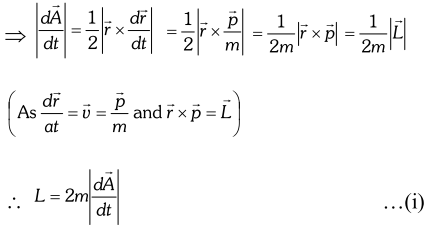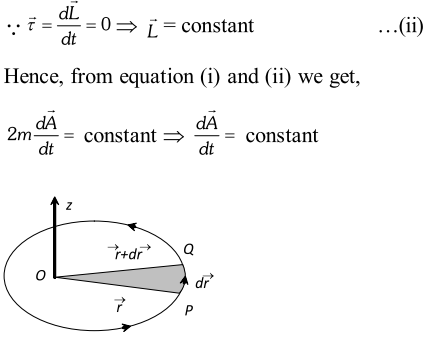Most Affordable JEE | NEET | 8,9,10 Preparation by Kota's Top IITian Doctor Faculties

# Important Questions of Gravitation Class 11 Physics: Fully Solved`

Get important questions of Gravitation Class 11 with their answers. View 11th Physics important questions for exam point of view. These important questions of gravitation will play significant role in clearing concepts of Physics class 11 as well as in revision of 12th students. This question bank is designed by NCERT keeping NCERT in mind and the questions are updated with respect to upcoming Board exams. You will get here all the important questions of other chapters along with other chapters of class 11 Physics with their proper solutions. So in this article we have provided solved important questions of Gravitation chapter that will help class 11 and 12 students. Click Here for Detailed Notes of Gravitation along with other subjects and chapters.

Q. Do the force of friction and other contact force arise because of gravitational force? If not, what is the origin of these forces?
Ans.The force of friction or any other contact forces, may be quite large and so it does not arise because of weak gravitational attraction. Such forces have electrical origin.
Q. Charge can be shielded from electrical forces by putting it inside a hollow conductor. Can a body be shielded from gravitational influence of nearby matter by putting it inside hollow sphere or by some other means?
Ans. No, a body can not be shielded from the gravitational field of nearby matter by any method.
Q.  The value of g on moon is $1 / 6$ th of that of earth. If a body is taken from earth to moon, then what will the change in its weight, Inertial mass and Gravitational mass.
Ans. The weight of body on moon will be $1 / 6$ th of that on the earth, but there will be no change in gravitational mass and inertial mass.

Q.  If the earth stops rotating about its axis, what will be the effect on the value of $^{\circ} \mathrm{g}^{\prime} ?$ Will this effect be same at all places?
Ans. The value of will increase at all places except at the poles. The increase will be different at different places, maximum at equator.

Q.  Can we determine the gravitational mass of a body inside on artificial satellite?
Ans. No, artificial satellite is like a freely falling body so that $g=0 .$

Q. The astronauts in a satellite orbiting the earth feel weightlessness. Does the weightlessness depend upon the distance of satellite from earth?
Ans.  No, the gravitational acceleration of the astronaut w.r.t. satellite is zero, whatever be the distance of the satellite from earth.

Q. If the kinetic energy of a satellite revolving in an orbit close to earth happens to be doubled, will the satellite escape?
Ans. The velocity of satellite will become equal to the escape velocity, hence it will escape.

Q. When an object falls to the ground, the earth moves up to it. Why is earth's motion not noticeable?
Ans. Because action and reaction are equal and opposite, hence the force with which earth is attracted towards the object is equal to the force with which earth attracts the object. $(-m g, m$ is the mass of the object). If $a^{\prime}$ be the acceleration produced in earth and $M$ is the mass of earth, then $a=\frac{m g}{M}$ As $M>>m,$ hence $a< Q. An artificial satellite revolves in its orbit around the earth without using any fuel. But aeroplane requires fuel to fly at a certain height. Why so? Ans. Air is required for aeroplane to fly hence the aeroplane requires fuel against the frictional force due to air. On the other hand, the satellite orbits at a much more height where almost no air is present and its friction in negligible. Q. An elephant and an ant are to be projected out of earth into space. Do we need different velocities to do so? Ans. The escape velocity$v_{e}=\sqrt{2 g R}$does not depend upon the mass of the projectile. Thus we need the same velocity to project an elephant and an ant into space. Q. Why are space rockets usually launched from west to east in the equatorial plane? Ans. since earth rotates from west to east and as such all points on the earth have velocity from west to east. Moreover, this velocity is maximum in the equatorial plane as . This maximum velocity is added to the launching velocity of the rocket and hence launching becomes easier. Q. Does a rocket really need the escape velocity of$11.2 \mathrm{km} / \mathrm{s}$initially to escape from the earth? Ans. No, rocket can have any velocity at the start. The rocket can continue to increase the velocity due to thrust provided by the escaping gases that will carry it to a desired position. #### India's Best Exam Preparation for Class 11th - Download Now Q. Does a planet revolving around sun in an elliptical orbit have a constant (i) Linear speed (ii) Angular momentum (iii) Kinetic energy (iv) Potential energy (v) Total energy throughout its orbit Ans. (i) According to the law of conservation of angular momentum, the planet moves faster when it is close to the sun and moves slower when it is farther away from the sun. Hence linear speed of the planet does not remain constant. (ii) According to the law of conservation of angular momentum, the angular momentum of planet remains constant. (iii) Since linear speed of the planet around the sun changes, therefore, its kinetic energy also changes continuously. (iv) Potential energy depends upon the distance between sun and planet, hence potential energy changes as the distance between the sun and planet changes continuously. (v) Total energy of planet remains constant. Q. In a spaceship moving in gravity free region, the astronaut will not be able to distinguish between up and down. Explain. Ans. The upward and downward sense is due to gravitational force of attraction between the body and the earth. In spaceship gravitational force is counter balanced by the centripetal force needed by satellite to move around the earth in circular orbit. Hence in absence of force, the astronaut will not be able to distinguish between up and down. Q. What do you understand by geo-stationary and polar satellites. Discuss their important uses. Ans. Geo-stationary or Geo-synchronous satellite : A satellite which appears stationary to an observer on earth is called geo-stationary satellite. Obviously, the velocity of such satellite relative to earth is zero. Clearly time of revolution of satellite hrs. This satellite is also called geo-synchronous satellite as its angular velocity is synchronised with angular speed of earth about its axis. i.e. this satellite revolves around the earth with same angular speed in same direction as is done by earth around its axis.Essential conditions for geo-stationary satellite: (a) A geo-stationary satellite should be at a height of nearly$36,000 \mathrm{km}$above the equator of earth. (b) The period of revolution around the earth should be the same as that of earth about its axis i.e. exactly 24 hours. (c) It should revolve in an orbit concentric and co- planer with the equatorial plane, so the plane of orbit of the satellite in normal to the axis of rotation of the earth. (d) Its sense of rotation should be same as that of earth about its own axis i.e. from west to east. Its orbital velocity is nearly 3.1$k m /$sec. Uses : Geo-stationary satellites are used for purposes of communications as they act as reflectors of suitable waves carrying the messages. Hence they are also called telecommunication satellites. India's INSAT-$2 \mathrm{B}, 2 \mathrm{C}$are communication satellites. Polar satellites : Such satellite which revolves in polar orbit around the earth is called polar satellites. A polar orbit is one whose angle of inclination with equatorial plane of earth is$90^{\circ}$and a satellite in polar orbit passes over the both north and south geographic poles respectively once in orbit. A single polar satellite can monitor$100 \%$earth's surface. Every locations on earth lies within the observation of polar satellite twice each day. These satellites are used for remote sensing and hence are called remote sensing satellites. Uses : In India remote sensing satellites (IRS-IA, IRS- -$1 \mathrm{B},$IRS-$1 \mathrm{C}$) are being used to collect the data for following purposes (i) For ground water survey. (ii) For detection the areas under forest. (iii) For preparing wasteland maps.#### India's Best Exam Preparation for Class 11th - Download Now (iv) For assessment of drought. (v) For the assessment of crop diseases. (vi) For detecting the potential fishing zones. (vii) For identifying the sources of pollution. (viii) To locate the position and movement of the troops of enemy and to locate the place and time for any nuclear explosion etc. i.e. for spying purposes. Q. State Kepler's laws of planetary motion and explain the deduction of Kepler's second law and third law of planetary motion. Ans. On the basis of his investigations, Kepler formulated the following three laws of planetary motion: (i) First law (law of orbit): Every planet revolves around the sun in an elliptical orbit, keeping sun at one focus of ellipse. (ii) Second law (Law of area): The radius vector drawn from sun to a planet sweeps out equal areas in equal intervals of time. i.e. areal velocity of the planet around the sun is constant. (iii) Third law (Law of period): The square of time period of revolution of a planet around the sun is directly proportional to the cube of semi major axis of its elliptical orbit i.e.$T^{2} \propto R^{3}$Deduction of second law: Let a particle rotates in$X-Y$plane about$z$-axis. At any time$t, \overrightarrow{O P}=\vec{r},$be the position vector of particle. The area swept by the position vector in small time$d t,|d \vec{A}|=$area of$\Delta O P Q=\frac{1}{2}|\vec{r} \times d \vec{r}|$since, a planet revolves around the sun, in an elliptical orbit under the influence of gravitational pull of sun on planets and this pull (or force) acts along the line joining the centres of the sun and theplanet and is directed towards the sun. Hence, the torque acting on planet is zero.i.e. areal velocity of the planet around the sun is constant, which is second law of planetary motion. Deduction of third law : Let a planet of mass$^{\circ} \mathrm{m}^{\prime}$is orbiting around the sun of mass$M$in a circular orbit of radius$^{\circ} r^{\prime}$with constant angular velocity$\omega,$then,$\frac{G M m}{r^{2}}=m \omega^{2} r=m\left(\frac{2 \pi}{T}\right)^{2} r \Rightarrow T^{2}=\left(\frac{4 \pi^{2}}{G M}\right) r^{3}$i.e.$T^{2} \propto r^{3} \quad\left(\because \frac{4 \pi^{2}}{G M}=\text { constant }\right)$Above result holds equally good for elliptical orbit provided we replace$r$with$^{*} a^{\prime},\$ the semi major axis of ellipse.
eSaral provides you complete edge to prepare for Board and Competitive Exams like JEE, NEET, BITSAT, etc. We have transformed classroom in such a way that a student can study anytime anywhere. With the help of AI we have made the learning Personalized, adaptive and accessible for each and every one. Visit eSaral Website to download or view free study material for JEE & NEET. Also get to know about the strategies to Crack Exam in limited time period.